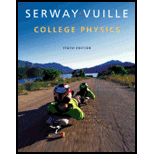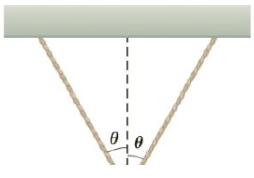# Two identical strings making an angle of θ = 30.0° with respect to the vertical support a block of mass m = 15.0 kg (Fig. P4.32). What is the tension in each of the strings?### College Physics

10th Edition
Raymond A. Serway + 1 other
Publisher: Cengage Learning
ISBN: 9781285737027

#### Solutions

Chapter
Section### College Physics

10th Edition
Raymond A. Serway + 1 other
Publisher: Cengage Learning
ISBN: 9781285737027
Chapter 4, Problem 6WUE
Textbook Problem
1887 views

## Two identical strings making an angle of θ = 30.0° with respect to the vertical support a block of mass m = 15.0 kg (Fig. P4.32). What is the tension in each of the strings?To determine
The tension on each of the strings.

### Explanation of Solution

Given info: The mass of the block is 15.0 kg. Strings make an angle of 30ο with respect to the vertical support.

The horizontal sine components of tension cancel each other because they are equal in magnitude and opposite in direction. The vertical cosine components get added to balance the weight of the block

From the above diagram,

2Tcosθ=mg

• m is the mass of the block.
• g is the acceleration due to gravity.
• T is the tension on the string.

On re-arranging the terms,

T=mg2cosθ

Substitute 15.0 kg for m, 30ο for θ and 9

### Still sussing out bartleby?

Check out a sample textbook solution.

See a sample solution

#### The Solution to Your Study Problems

Bartleby provides explanations to thousands of textbook problems written by our experts, many with advanced degrees!

Get Started

Find more solutions based on key concepts
Describe the three major soil-forming regimes.

Fundamentals of Physical Geography

State the law of conservation of mass. Describe how you might demonstrate this law.

General Chemistry - Standalone book (MindTap Course List)

For managing type 2 diabetes, regular physical activity can help by redistributing the body's fluids. T F

Nutrition: Concepts and Controversies - Standalone book (MindTap Course List)

Review. As shown in Figure P8.26, a light string that does not stretch changes from horizontal to vertical as i...

Physics for Scientists and Engineers, Technology Update (No access codes included)

Why do these dinoflagellates have a brownish-red color?

Oceanography: An Invitation To Marine Science, Loose-leaf Versin